# KSEEB Solutions for Class 8 Maths Chapter 11 Mensuration Ex 11.3

## KSEEB Solutions for Class 8 Maths Chapter 11 Mensuration Ex 11.3

Question 1.
There are two cuboidal boxes as shown in the adjoining figure. Which box requires the lesser amount of material to make?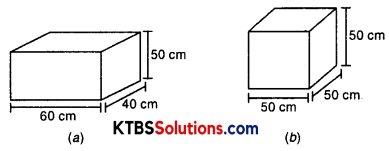Solution:
(a) Length of cuboid (l) = 60 cm
Breadth of cuboid (b) = 40 cm
Height of cuboil (h) = 50 cm
Anount of material required = Total surface area of cuboid
= 2(lb + bh + lh)
= 2(60 × 40 + 40 × 50 + 60 × 50)
= 2[2400 + 2000 + 3000]
= 2 × 7400
= 14800 cm2
Side of cube = 50 cm
(b) Total material required = Total surface area of cube
= 6(side)2
= 6(50)2
= 6 × 2500
= 15000 cm2
Cuboidal boxes required a lesser amount of material to make.Question 2.
A suitcase with measures 80 cm × 48 cm × 24 cm is to be covered with a tarpaulin cloth. How many meters of tarpaulin of width 96 cm is required to cover 100 such suitcases?
Solution:
Measurement of suitcase = 80 cm × 48 cm × 24 cm
Surface area of suitcase = 2(lb + bh + lh)
= 2[80 × 48 + 48 × 24 + 80 × 24]
= 2[3840 + 1152 + 1920]
= 2
= 13824 cm2
Area of cloth required for 100 suitcases = 100 × 13824 = 1382400 cm2
Area of cloth = l × b = l × 96
l × 96 = 1382400
l = $$\frac{1382400}{96}$$ = 14400 cm (approx.)
or length of cloth = $$\frac{14400}{100}$$ m = 144 mts.

Question 3.
Find the side of a cube whose surface area is 600 cm2.
Solution:
Surface area of cube = 600 cm2
⇒ 6(Side)2 = 600
⇒ (Side)2 = $$\frac{600}{6}$$ = 100
⇒ Side = √100 = 10 mtsQuestion 4.
Rukhsar painted the outside of the cabinet of measure 1 m × 2 m × 1.5 m. How much surface area did she cover if she painted all except the bottom of the cabinet.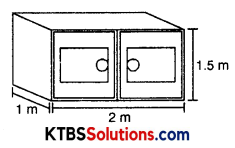Solution:
Measurement of cabinet = 1 m × 2 m × 1.5 m
Surface area of cabinet except base = 2 (lb + bh + lh) – lb
= 2[1 × 2 + 2 × 1.5 + 1.5 × 1] – 1 × 2
= 2[2 + 3 + 1.5] – 2
= 2[6.5] – 2
= 13 – 2
= 11 cm2

Question 5.
Daniel is painting the walls and ceiling of a cuboidal hall with length, breadth, and height of 15 m, 10 m, and 7 m respectively. From each can of paint 100 m2 of the area is painted. How many cans of paint will she need to paint the room?
Solution:
Length of hall = 15 m
The breadth of hall = 10 m
Height of hall = 7 m
Area of wall and ceiling of cuboidal hall = 2(l + b)h + lb
= 2[15 + 10] × 7 + 15 × 10
= 2 × 7 + 150
= 350 + 150
= 500 m2
1 m2 is painted by = 1 can
1 m2 is painted by = $$\frac{1}{100}$$
500 m2 is painted by = $$\frac{1}{100}$$ × 500 = 5 cans.Question 6.
Describe how the two figures at the right are alike and how they are different. Which box has a larger lateral surface area?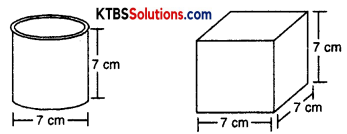Solution:
They are alike, both have the same height. They are different as one is cylindrical in shape and other is cubical.
Lateral surface area of cylinder = 2πrh
= $$2 \times \frac{22}{7} \times \frac{7}{2} \times 7$$
= 154 cm2
Lateral surface area of cube = 4(side)2
= 4(7)2
= 4 × 49
= 196 cm2

Question 7.
A closed cylindrical tank of radius 7 m and height 3 m is made from a sheet of metal. How many sheets of metal are required?
Solution:
Radius of cylinder = 7 cm
Height of cylinder = 3 m
Total surface area of cylinder = 2πr(r + h)
= 2 × $$\frac{22}{7}$$ × 7[7 + 3]
= 44 × 10
= 440 m2
Hence, 440 m2 of metal sheet is required to make the closed cylindrical tank.Question 8.
The lateral surface area of a hollow cylinder 4224 cm2. It is cut along its height and formed a rectangular sheet of width 33 cm. Find the perimeter of the rectangular sheet?
Solution:
Lateral surface area of hollow cylinder = 4224 cm2
Width of rectangular sheet = 33 cm
Area of sheet = LSA of cylinder
⇒ l × b = 4224
⇒ l × 33 = 4224
⇒ l = $$\frac{4224}{33}$$ = 128 cm
Perimeter of rectangular sheet = 2(l + b)
= 2(128 + 33)
= 2 × 161
= 322 cm

Question 9.
A road roller takes 750 complete revolutions to move once over to level a road. Find the area of the road if the diameter of a road roller is 84 cm and the length is 1 m.
Solution:
Length of roller = 1 m
Radius of roller = $$\frac{84}{2}$$ = 42 cm or $$\frac{42}{100}$$ = 0.42 m.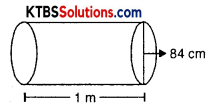Area covered in 1 revolution = 2πrh
= 2 × $$\frac{22}{7}$$ × 0.42 × 1
= 44 × 0.06
= 2.64 m2
Area covered in 750 revolution = 750 × 2.64 = 1980 m2
∴ Area of road = 1980 m2Question 10.
A company packages its milk powder in a cylindrical container whose base has a diameter of 14 cm and height of 20 cm. The company places a label around the surface of the container (as shown in the figure). If the label is placed 2 cm from top and bottom, what is the area of the label?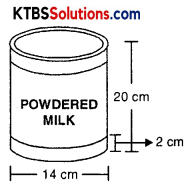Solution:
Height of cylindrical container = 20 cm
Label is placed 2 cm from top and bottom.
Height of label = 20 – (2 + 2)
= 20 – 4
= 16 cm
Radius of cylinder = 7 cm
Area of label = 2πrh
= 2 × $$\frac{22}{7}$$ × 7 × 16
= 44 × 16
= 704 cm2